## Icosidodecahedron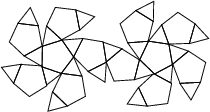An Archimedean Solid whose Dual Polyhedron is the Rhombic Triacontahedron. It is one of the two convex Quasiregular Polyhedra and has Schläfli Symbol. It is also Uniform Polyhedronand has Wythoff Symbol. Its faces are. The Vertices of an icosidodecahedron of Edge lengthare,,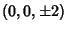,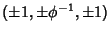,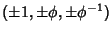,. The 30 Vertices of an Octahedron 5-Compound form an icosidodecahedron (Ball and Coxeter 1987). Faceted versions include the Small Icosihemidodecahedron and Small Dodecahemidodecahedron.

The faces of the icosidodecahedron consist of 20 triangles and 12 pentagons. Furthermore, its 60 edges are bisected perpendicularly by those of the reciprocal Rhombic Triacontahedron (Ball and Coxeter 1987).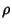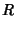See also Archimedean Solid, Great Icosidodecahedron, Quasiregular Polyhedron, Small Icosihemidodecahedron, Small Dodecahemidodecahedron

References

Ball, W. W. R. and Coxeter, H. S. M. Mathematical Recreations and Essays, 13th ed. New York: Dover, p. 137, 1987.

Wenninger, M. J. Polyhedron Models. Cambridge, England: Cambridge University Press, p. 73, 1989.